Smartick is an advanced online program that teaches kids math and coding in only 15 min. a day

Apr16

# Using the Number Line to Compare Fractions

In previous posts, we’ve learned how to place different numbers on the number line. Today we’re going to learn how to represent fractions on a number line. This is very useful when we need to compare them.

#### Fractions with the same denominator

As we know, it’s easy to compare fractions with the same denominator. In these cases, the fraction with the larger numerator is the bigger fraction. For example, eight-thirds is bigger than three thirds.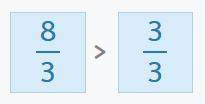#### Fractions with the same numerator

Something that seems a bit more complicated, but only on the surface, is comparing fractions with the same numerator. In this situation, the fraction with the smaller denominator is always the bigger fraction. For example, fifteen-thirds is bigger than fifteen fourths.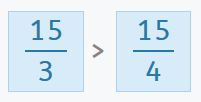#### Using the number line for fractions with different numerators and denominators

The comparison becomes more complicated when the fractions we’re comparing don’t have the same numerator or denominator. Which fraction is greater: eleven thirds or thirteen fourths?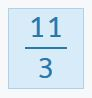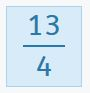This is where the number line is particularly useful. We can use it to give us a visual representation of the fractions, which we can then compare without having to do any mathematical calculations. Although the number of subdivisions of each unit will be different on the number line for each fraction, it’s important to make sure the unit is the same size on each one. Then we can compare the fractions visually.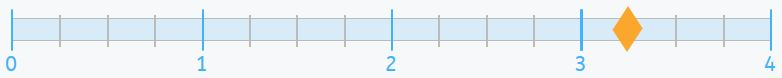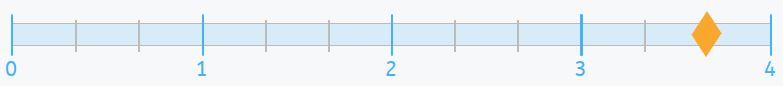Once we’ve placed the two fractions with different numerators and denominators on the number line, we can tell which of the two is the greater simply by looking. Without having to make any calculations or operate with fractions, we can see that thirteen-fourths is less than eleven thirds. The first fraction is placed close to the three, while the second fraction is closer to the four.

It’s easy, right?